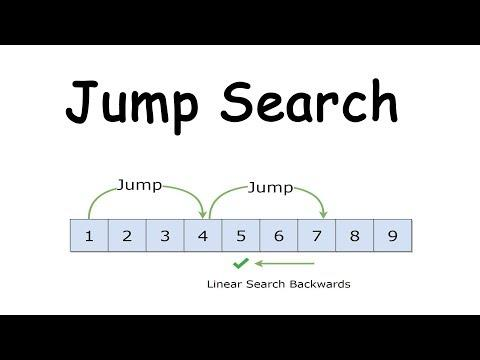# Jump Search

Searching and sorting is always one of the main thing to know and improve about. Like sorting, searching also get special attention from the computer scientists. Today we will learn about a special searching algorithm named Jump sort.

### Jump Search

In the vast world of computation, jump search refsembles to a search algorithm for ordered lists. It functions by first checking all items at the edge of block item where m is the size of the block. When an item is found that is larger than the search key, then we do the linear size to find the exact location of the item. The optimal value of m is √n, where n is the length of the list L. Because both steps of the algorithm look at, at most, √n items the algorithm runs in O(√n) time. This number signifies its betterness than linear search and worse than binary search. The main merit over the binary search is that a jump search only needs to jump backwards once, while a binary can jump backwards up to log n times. This can be a key point if a jumping backwards takes significantly more time than jumping forward.

### Algorithm

Below is the general algorithm for jump search implementation:

Input: sorted array A with n elements

Output: The index of searched item or -1

The steps for this mechanism are:

1. Set the starting index, i to 0 and step size,m to √n.

2. Make a comparison between A[i] with item. If A[i] != item and A[i] < item, then jump to the next block. Also, do the following:

``````Reset index, i = step size,m
Increment step size, m by √n
``````
3. Repeat the step 2 untill m comes to the end of given array.

4. If A[i] > item, then move to the beginning of the current block and perform a linear search.

``````Set start of linear search, x = i
Compare A[x] with item. If A[x]== item, then print x as the valid location else increment x by 1
``````
5. Continue this procedure untill x comes to the end of step block. and return -1 as not found### Implementation

Below is the ruby implementation of jump search

``````def jump_search array, item
n = array.size
i = 0
m = Math.sqrt n

while array[m] <= item && m < n do
i = m
m += Math.sqrt n
return -1 if m > n - 1
end
start = i

while start < m do
return x if a[x] == item
start += 1
end
-1
end
``````

### Complexity

We determine the time and space complexity in the following:

#### Time Complexity:

The while loop in the above code executes `n/m` times as the loop counter increments by m times in every iteration. Since the optimal value of m= √n , thus, n/m=√n resulting in a time complexity of O(√n).

#### Space Complexity:

The space complexity of this algorithm is O(1) since it does not requireany other data structure for its implementation.

### More Optimal Jump Search

Certainly the execution time of this algorithm is O(√n), There is an improvement section for this algorithm too. if we carefully see, once we know the interval where the value is we can optimize it by applying jump search again on that interval. For example let’s say the list length is 1,000. The jump interval should be: √1000=32. Since we can see closely, we can use jump search with a new step √32≈6. Every time we find the desired interval we can adopt the jump search algorithm with more smaller step. Finally the step will be 1. In that case the complexity of the algorithm does not stuck to O(√n). Now its complexity is more close to logarithmic value. The drawback of this approach is that the implementation of this approach is considered to be more difficult than the binary search, where the complexity is also O(log(n)).

Although it is not netter than binary search in terms of complexity and cannot work without sorted list, still i think it is good one to learn and apply in applicable space. So you can study more if you get some interest. Thank you